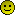###Author Topic: WireWorld in 13 lines of code  (Read 2308 times)

0 Members and 1 Guest are viewing this topic.

####rain_storm

• Here comes the Rain
••• Posts: 3088
• Karma: 182
• Rain never hurt nobody##### WireWorld in 13 lines of code
« on: August 17, 2011 »
WireWorld is a cellular automaton that can simulate electronic components such as Logic Gates. And since Logic Gates are the building blocks of CPU's it's no wonder that some geeks have already built a CPU in WireWorld.

Here is a WireWorld simulator written in 13 lines of ps2yabasic source code. With the limitation that every single line of code should fit within the default size of the emulators editor display, colons are allowed to separate statements within a single line. The horizontal scroll bar remains inactive so I don't make use of that final column either. There isn't much wasted space at the end of the lines so you'd be hard pressed to shave off some lines of code.

controls:
up,down,left,right = move the cursor
cross = toggle state of the selected cell (from void to wire to tail to head)
triangle,circle,square = run the simulator

Code: [Select]
`open window 1,1:data 64,51,10,16,32,64,128,4096,8192,16384,32768 data 255,255,0,0,0,127,127,127,255:dim C(641,513),R(3),G(3),B(3) read W,H,S,U,R,D,L,T,O,C,Q:for i=1 to 3 read R(i),G(i),B(i) next 0:setdispbuf B:B=1-B:setdrawbuf B:for y=1 to H for x=1 to W      c=C(x,y):setrgb 1,R(c),G(c),B(c):fill box x*S,y*S,x*S-S,y*S-S    next next:setrgb 1,99,0,0:box X*S,Y*S,X*S-S,Y*S-S:P=peek("port1")if(and(P,C)=C)C(X,Y)=and(C(X,Y)+1,3):X=X+and(P,R)/R-and(P,L)/L:  X=min(max(X,1),W):Y=min(max(Y+and(P,D)/D-and(P,U)/U,1),H):F=Q+T+Oif(and(P,F)=0)goto 0:dim T(W,H):for y=1 to H for x=1 to W:N=0:   for v=y-1 to y+1 for u=x-1 to x+1 if(C(u,v)=3)N=N+1 next next:   T(x,y)=1:if(C(x,y)=0)then T(x,y)=0 elsif(C(x,y)=2)then T(x,y)=1  elsif(C(x,y)=3)then T(x,y)=2 elsif(N=1 or N=2)then T(x,y)=3 fi   next next:for y=0 to H for x=0 to W C(x,y)=T(x,y)next next:goto 0`

####Shockwave

• good/evil
• Founder Member
••That's excellent RainStorm